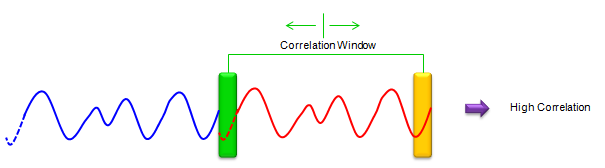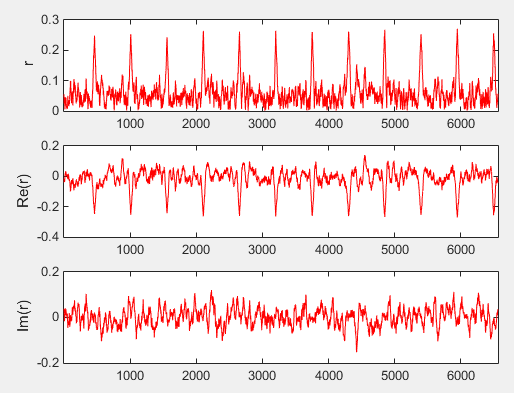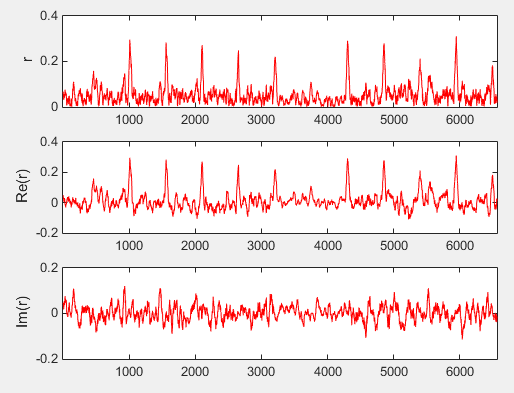LTE Quick Reference LTE Timing Sync : Cyclic Prefix Correlation   This is about finding the symbol boundary (the start of a OFDMA symbol) by using the fact that Cyclic Prefix is an exact copy of the last part of OFDM symbol. Therefore, the correction between the cyclic prefix and the ending part of an OFDM symbol should be very high as illustrated below (Refer to OFDM : Cyclic Prefix part for the details). If we use this property, we may find the location of a Cyclic prefix. Once you locate the Cyclic Prefix, you can locate the start of the OFDM Symbol automatically. This method may not be very accurate depending on situation. So we would not use this method only to find the start of a symbol, but this can be a good rough estimate and very simple to implement.Following is a sample Matlab code to calcualting correction with the length of cyclic prefix sliding along the I/Q data sequence. clear all;     fid = fopen('UL_5_25_NoFilter_S7_68_Trig.bin','r'); [data,count] = fread(fid, 'single'); fclose(fid);   Offset = 280228 + 2 ;   Nfft = 512; SamplingScale = (double(Nfft)/2048); CP_LengthList = SamplingScale * [160;144;144;144;144;144;144]; Symbol_LengthList = SamplingScale * [2048;2048;2048;2048;2048;2048;2048];   dataI = data(1:2:end); dataQ = data(2:2:end); dataComplex = dataI + j*dataQ;   rSeq = []; rThreshold = 0.2; rPeakIndex = []; NoOfSymbolsToScan = 10;   SearchStart = 0;   for i = (SearchStart+0):(SearchStart + NoOfSymbolsToScan*Nfft + NoOfSymbolsToScan*144)     X = dataComplex(i+1:i+144/4);     Y = dataComplex(i+1+Nfft:i+Nfft+144/4);     r = X' * Y;     rSeq = [rSeq r]; end;   pks = find(abs(rSeq) > 0.24);   subplot(3,1,1); plot(abs(rSeq),'r-'); xlim([1 length(rSeq)]); ylabel('r');   subplot(3,1,2); plot(real(rSeq),'r-'); xlim([1 length(rSeq)]);ylabel('Re(r)');   subplot(3,1,3); plot(imag(rSeq),'r-'); xlim([1 length(rSeq)]);ylabel('Im(r)');     From an uplink IQ sample data (fid = fopen('UL_5_25_NoFilter_S7_68_Trig.bin','r') ), I got following result. (If you want to try this code with the same data that I used, download the data from the link)From an uplink IQ sample data (fid = fopen('LTE_DL_5M_25RB_S_7_68_SG_Trig.bin','r')), I got following result. (If you want to try this code with the same data that I used, download the data from the link)